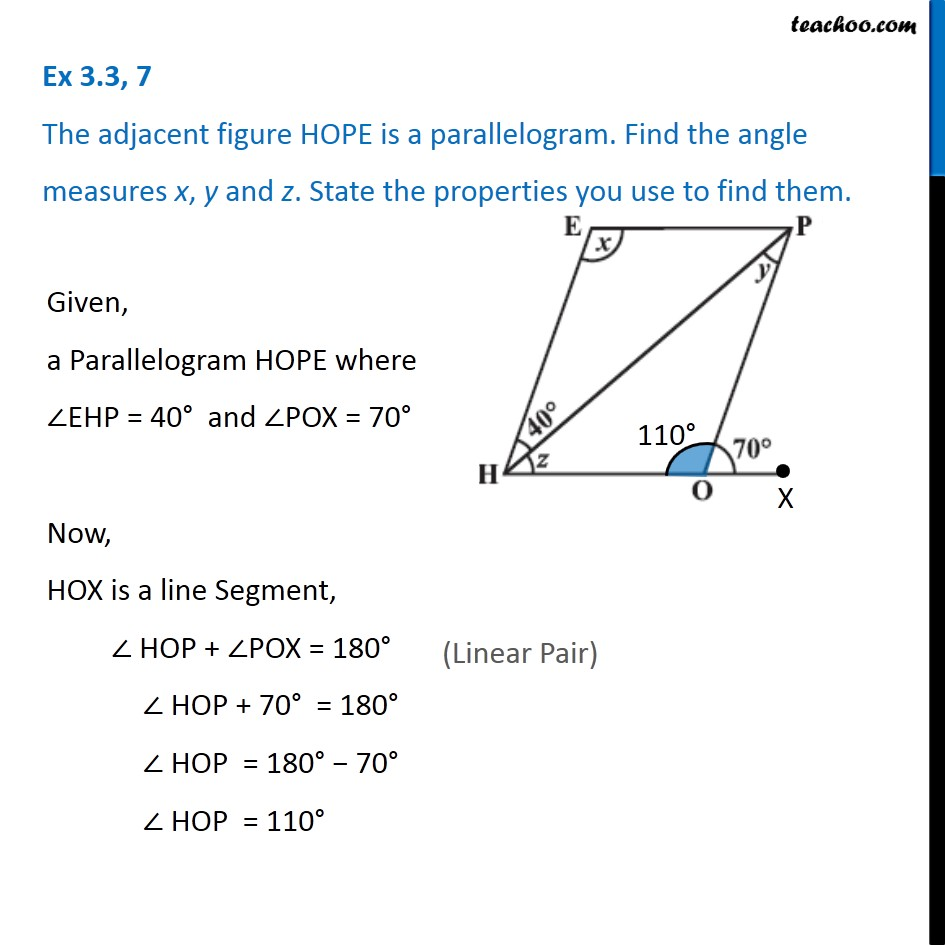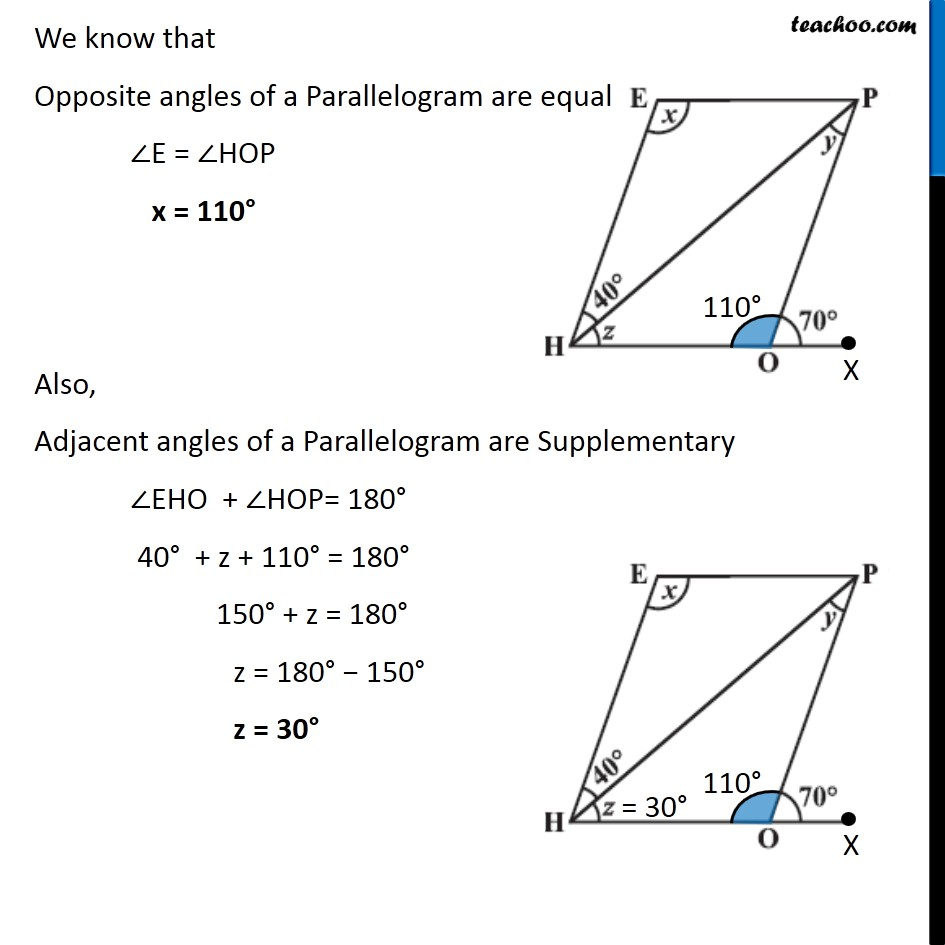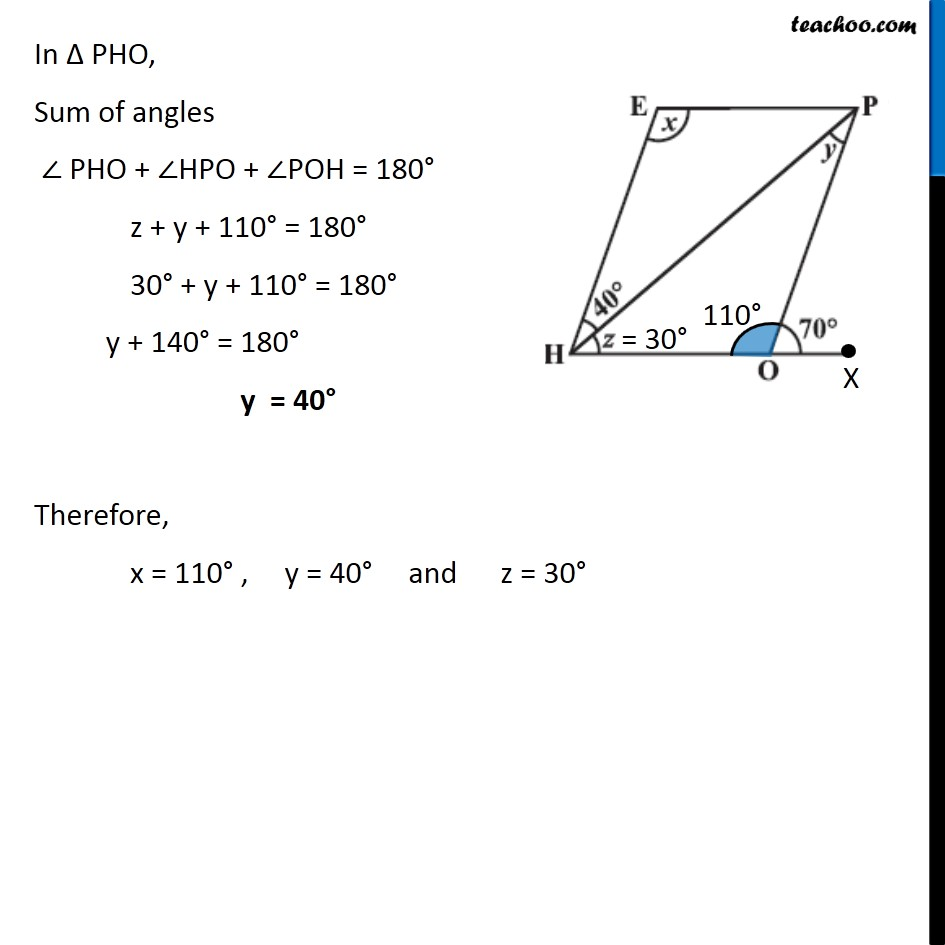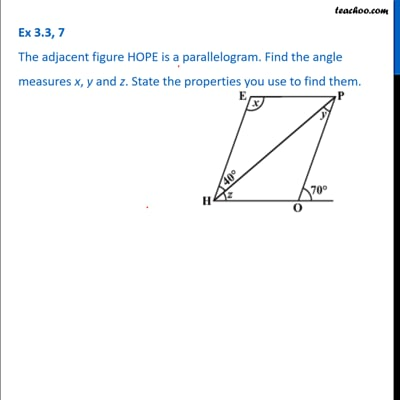Ex 3.3

Chapter 3 Class 8 Understanding Quadrilaterals
Serial order wiseThis video is only available for Teachoo black users

### Transcript

Ex 3.3, 7 The adjacent figure HOPE is a parallelogram. Find the angle measures x, y and z. State the properties you use to find them. Given, a Parallelogram HOPE where ∠EHP = 40° and ∠POX = 70° Now, HOX is a line Segment, ∠ HOP + ∠POX = 180° ∠ HOP + 70° = 180° ∠ HOP = 180° − 70° ∠ HOP = 110° We know that Opposite angles of a Parallelogram are equal ∠E = ∠HOP x = 110° Also, Adjacent angles of a Parallelogram are Supplementary ∠EHO + ∠HOP= 180° 40° + z + 110° = 180° 150° + z = 180° z = 180° − 150° z = 30° In ∆ PHO, Sum of angles ∠ PHO + ∠HPO + ∠POH = 180° z + y + 110° = 180° 30° + y + 110° = 180° y + 140° = 180° y = 40° Therefore, x = 110° , y = 40° and z = 30°